The impact of distance metrics on K-means clustering algorithm using in network intrusion detection data

Đăng ngày 4/2/2019 4:00:01 PM | Thể loại: | Lần tải: 0 | Lần xem: 7 | Page: 4 | FileSize: 0.22 M | File type: PDF
The impact of distance metrics on K-means clustering algorithm using in network intrusion detection data. This paper aimed to evaluate the impact of Euclidean and Manhattan distance metrics on Kmeans algorithm using for clustering KDD cup99 intrusion detection data. Experimental results indicate that Manhattan distance metric performs better in terms of performance evaluation metrics than Euclidean distance metric.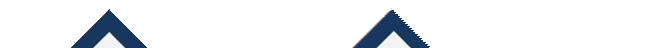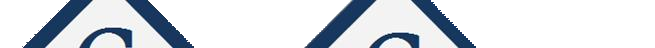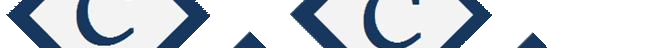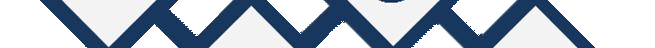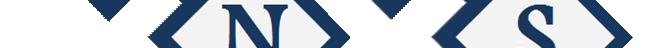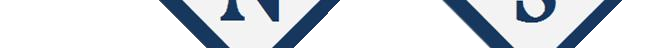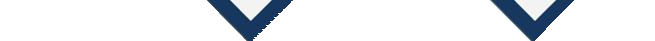International Journal of Computer Networks and Communications Security
VOL. 3, NO. 5, MAY 2015, 225–228
Available online at: www.ijcncs.org
E-ISSN 2308-9830 (Online) / ISSN 2410-0595 (Print)
The Impact of Distance Metrics on K-means Clustering Algorithm
Using in Network Intrusion Detection Data
FARRAHI4
1, 2, 3, 4 Shiraz University of Technology, Department of Computer Engineering and IT, Shiraz, Iran
ABSTRACT
A Network Intrusion Detection System (NIDS) can detect suspicious activities that aimed to harm the
network. Since, NIDS help us to keep the networks safer many researchers are motivated to propose more
accurate NIDS. K-means clustering algorithm is a distance-based algorithm which widely used in IDS
research area. This paper aimed to evaluate the impact of Euclidean and Manhattan distance metrics on K-
means algorithm using for clustering KDD cup99 intrusion detection data. Experimental results indicate
that Manhattan distance metric performs better in terms of performance evaluation metrics than Euclidean
distance metric.
Keywords: K-means Clustering, Network Intrusion Detection, Euclidean Distance, Manhattan Distance,
Data Mining.
1
INTRODUCTION
Network
anomaly
detection
is
the
act
of
In computer security a threat is a possible danger
identifying
intrusive
behaviors
in
the
computer
that might use the system vulnerabilities in order to
networks. Data mining techniques can improve the
harm the system. An Intrusion Detection System
accuracy and false alarm rate of Network-based
(IDS) can detect suspicious activities that aimed to
IDS (NIDS). Clustering techniques are one of the
harm the network. So, an IDS can help network
most important data mining techniques that work
and
organizations
to
keep
their
with unlabeled data . Clustering techniques are
networks safer. Up to now, the researchers are
appropriate for Network anomaly detection since,
motivated
in
network
and
information
security
they can handle unlabeled data.
research area  because computer networks have
Clustering algorithms group the data base on
many vulnerabilities and IDS can protect them from
their similarities. In other words, the data which are
intruders.
grouped in the same cluster are similar to each
IDS can be classified into two major categories
other and dissimilar to the data which belong to
[2, 3]: Misuse-based Intrusion Detection System
other
clusters.
The
ability
of
the
clustering
(MIDS)
and
Anomaly-based
Intrusion
Detection
algorithms can help us in NIDS. Since, normal
System
(AIDS).
The
major
difference
between
behaviors and anomalous behaviors are different
these
two
categories
is
in
the
way
of
finding
from each other, clustering algorithms can group
intrusive
activities.
A
MIDS
tries
to
identify
normal behaviors in the same clusters and assign
intrusive behaviors based on the patterns that are
anomalous behaviors to the other clusters .
available in a database. As a MIDS has well known
In this paper, K-means clustering algorithm has
patterns in a database, its accuracy is high. An
been utilized to cluster network intrusion detection
AIDS tries to identify the intrusive behaviors that
data. It is proved that K-means clustering algorithm
significantly different from normal behaviors. An
has promising results in network intrusion detection
AIDS is less accurate than a MIDS but can detect
. K-means clustering algorithm needs a distance
previously unseen attacks.
metric for calculation of the distances between data
objects . This paper evaluated the impact of two1
n
n
226
H. Nasooti et. al / International Journal of Computer Networks and Communications Security, 3 (5), May 2015
different
distance
metrics
on
the
clustering
of
a user specified parameter. Since, K-means is a
network intrusion data.
simple algorithm it has been used in a wide variety
The remainder of this paper organized as follows:
of applications  as well as network intrusion
Section
2
presents
literature
review
.Section
3
detection. It is one of the most important clustering
explains K-means clustering algorithm and distance
algorithms in data mining area.
metrics .Section 4 presents the motivation of our
K-means algorithm is described as follows :
paper. Section 5 presents simulation parameters and
performance metrics. Finally, section 6 concludes
the paper and explains the results.
1)
2)
Choose K data objects for the cluster centers,
randomly.
Calculate the distances between each data
2
RELATED WORKS
object
and
all
cluster
centers
base
on
a
In  K-means clustering algorithm has been
used for network intrusion detection. Simulation
results show that K-means clustering algorithm is
able to detect unknown intrusions in the network
connections. Euclidean metric has been used as the
distance metric.
3)
4)
5)
distance metric.
Assign data objects to the closet cluster
center.
Update cluster centers.
Iterate steps (2)-(4) until there is no more
updating.
In  the authors proposed an algorithm based on
K-means clustering algorithm for network intrusion
detection. The proposed algorithm can define the
number of clusters automatically based on a
predefined threshold.
Y-Means  is a clustering algorithm for
network intrusion detection based on K-means
clustering algorithm. Y-means is able to define the
number of clusters automatically and overcome the
shortcoming of producing empty clusters in K-
means algorithm.
In  five different clustering algorithms
including K-means clustering algorithm and four
different classifiers have been evaluated for
network intrusion detection. The results show that
A distance metric must be defined for distance
calculation between data objects in K-means
algorithm. The most common distance metric that
uses in K-means clustering algorithm is Euclidean
distance metric. Although, Euclidean distance
metric is the most common metric, Manhattan
distance metric can be used in K-means algorithm.
Consider X, Y are two data objects which
described by n attributes. The Euclidean and
Manhattan distances between data objects X, Y are
denoted as Euclid(X,Y) and Manhat(X,Y),
respectively. xj and yj are the jth attribute values of
data objects X and Y, respectively. Euclidean and
Manhattan distance metrics are defined as follow:
the distance-based clustering algorithms are more
accurate in detecting unknown attacks in comparing
to the classifiers.
All the previous works used Euclidean distance
metric for calculation of the distances. None of the
previous works had not employed another distance
metric such as Manhattan distance in K-means
algorithm. This paper is aimed to analysis the
4
Euclid(X,Y) =[(xj yj )2 ]2
j=1
Manhat(X,Y) = | xj yj |
j=1
MOTIVATION
(1)
(2)
impact of different distance metrics on K-means
clustering algorithm using in network intrusion
detection. So, the impact of Manhattan and
Euclidean distance metrics on K-means algorithm
using in network intrusion detection has been
evaluated in this paper.
Almost, heuristic methods are used for The
distance metric is one of the key steps in the
unsupervised learning process . Many
parameters effect the final clustering results of K-
means algorithm such as, algorithm initialization
and distance metric. As changing the distance
3
K-MEANS CLUSTERING ALGORITHM
metric might change the final clustering results so,
it is important to evaluate the impact of other
Clustering is one the most important data mining
techniques that can handle unlabeled data. K-means
is a distance-based clustering algorithm. K-means
groups the data objects into K disjoint clusters. K is
distance metrics on K-means algorithm in
clustering network intrusion data. This paper is
aimed to analysis the impact of Euclidean and
Manhattan distance metrics on the application of
network intrusion detection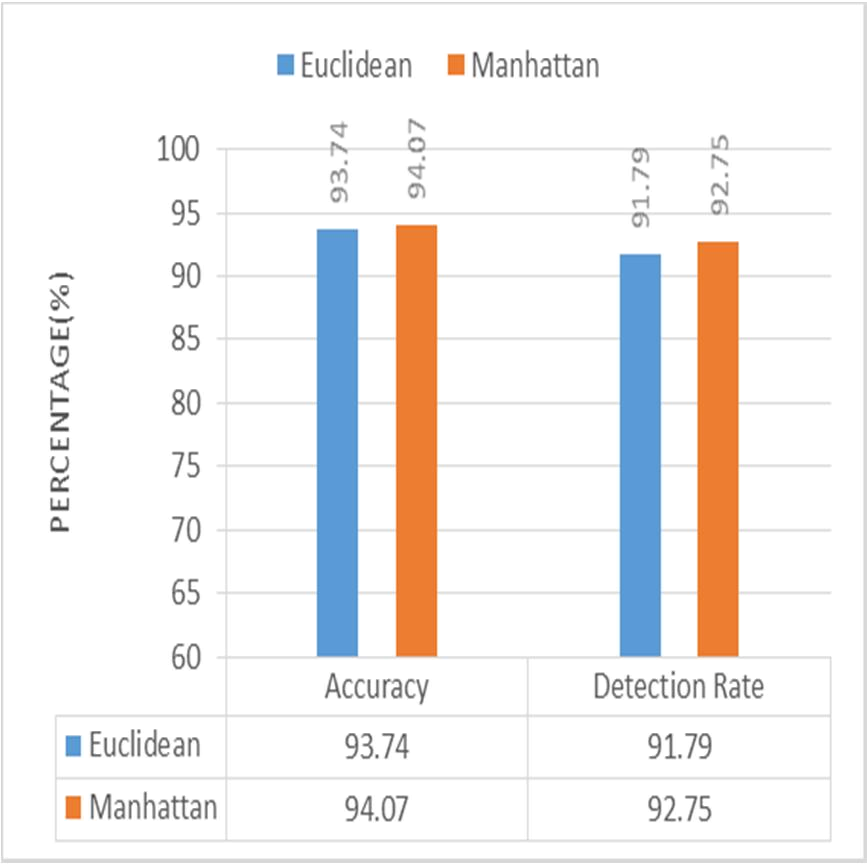X* =
227
H. Nasooti et. al / International Journal of Computer Networks and Communications Security, 3 (5), May 2015
5
SIMULATION DESIGN
False negative (FN): number of data objects
that are incorrectly classified as intrusion.
In
this
paper,
we
used
KDDcup
99
network
intrusion
data
subset
.
KDDcup
intrusion
6
RESULTS
detection data set consisted of 4 attack types plus
normal type activities. The four attack types are
Denial of Service (DoS), Remote to User (R2L),
User to Root (U2R) and Probing. 10% KDDcup 99
has 494,021 data objects. We sampled 72,784 data
objects for our experiments. The experiments have
been done with five clusters (K is five in our
The performance of Euclidean and Manhattan using
as distance metrics in K-means algorithm have been
shown in Fig. 1. Manhattan distance metric
performs better than Euclidean distance metric in
K-mean clustering algorithm in terms of accuracy
and detection rate.
experiments).
The data have been normalized based on Min-
Max method .
Formula 3 shows Min-Max
normalization method where MIN(i) and MAX(i)
are the minimum and
attribute, respectively.
maximum value of ith
X corresponds to an
unnormalized attribute value before transformation
and X* corresponds to the normalized value of X.
By using Formula 3 all data were scaled in the
range [0-1].
X MIN(i)
MIN(i) MAX(i)
(3)
5.1
Performance Evaluation Metrics
The
impact
of
Manhattan
and
Euclidean
distances metric in K-means algorithm have been
evaluated in terms of accuracy, detection rate and
false alarm rate. These performance metrics are as
Fig. 1. Comparison of Detection Rate and Accuracy
following:
between Euclidean and Manhattan Metrics.
Accuracy = (TP + TN)
(TP + TN + FP + FN)
(4)
The false alarm rate of Euclidean distance metric
is 5.23% and the false alarm rate of Manhattan
distance metric is 4.72%. It means that Manhattan
distance metric is superior to Euclidean distance
Detection Rate = (TP)
(TP + FP)
(5)
metric in term of false alarm rate.
Generally, Manhattan distance metric has better
performance than Manhattan distance metric using
in
K-means
algorithm
for
clustering
network
FalseAlarmRate = (FP)
(FP + TN)
(6)
intrusion detection data.
As a consequence, the impact of distance metrics
should be evaluated for other distance based
True positive (TP): number of attack data
objects that are correctly classified as
intrusion.
True negative (TN): number of normal data
objects that are correctly classified as
normal.
False positive (FP): number of normal data
objects that are incorrectly classified as
attacks.
algorithm using in network intrusion detection area
since, other distance metrics might have better
results.
Many researchers such as [6, 7] proposed
distance-based algorithms for network intrusion
detection but the authors only used Euclidean
distance metric for calculation of the distances.
Other distance metrics such as Manhattan metric
might have better results in the proposed
algorithms.

Bước 1:Tại trang tài liệu slideshare.vn bạn muốn tải, click vào nút Download màu xanh lá cây ở phía trên.
Bước 2: Tại liên kết tải về, bạn chọn liên kết để tải File về máy tính. Tại đây sẽ có lựa chọn tải File được lưu trên slideshare.vn
Bước 3: Một thông báo xuất hiện ở phía cuối trình duyệt, hỏi bạn muốn lưu . - Nếu click vào Save, file sẽ được lưu về máy (Quá trình tải file nhanh hay chậm phụ thuộc vào đường truyền internet, dung lượng file bạn muốn tải)
Có nhiều phần mềm hỗ trợ việc download file về máy tính với tốc độ tải file nhanh như: Internet Download Manager (IDM), Free Download Manager, ... Tùy vào sở thích của từng người mà người dùng chọn lựa phần mềm hỗ trợ download cho máy tính của mình
7 lần xem

Nội dung

International Journal of Computer Networks and Communications Security VOL. 3, NO. 5, MAY 2015, 225–228 Available online at: www.ijcncs.org E-ISSN 2308-9830 (Online) / ISSN 2410-0595 (Print) The Impact of Distance Metrics on K-means Clustering Algorithm Using in Network Intrusion Detection Data HADI NASOOTI1, MARZIEH AHMADZADEH2, MANIJEH KESHTGARY3 and S. VAHID FARRAHI4 1, 2, 3, 4 Shiraz University of Technology, Department of Computer Engineering and IT, Shiraz, Iran E-mail: 1nasooti.ha@gmail.com, 2ahmadzadeh@sutech.ac.ir ABSTRACT A Network Intrusion Detection System (NIDS) can detect suspicious activities that aimed to harm the network. Since, NIDS help us to keep the networks safer many researchers are motivated to propose more accurate NIDS. K-means clustering algorithm is a distance-based algorithm which widely used in IDS research area. This paper aimed to evaluate the impact of Euclidean and Manhattan distance metrics on K-means algorithm using for clustering KDD cup99 intrusion detection data. Experimental results indicate that Manhattan distance metric performs better in terms of performance evaluation metrics than Euclidean distance metric. Keywords: K-means Clustering, Network Intrusion Detection, Euclidean Distance, Manhattan Distance, Data Mining. 1 INTRODUCTION In computer security a threat is a possible danger that might use the system vulnerabilities in order to harm the system. An Intrusion Detection System (IDS) can detect suspicious activities that aimed to harm the network. So, an IDS can help network administrators and organizations to keep their networks safer. Up to now, the researchers are motivated in network and information security research area  because computer networks have many vulnerabilities and IDS can protect them from intruders. IDS can be classified into two major categories [2, 3]: Misuse-based Intrusion Detection System (MIDS) and Anomaly-based Intrusion Detection System (AIDS). The major difference between these two categories is in the way of finding intrusive activities. A MIDS tries to identify intrusive behaviors based on the patterns that are available in a database. As a MIDS has well known patterns in a database, its accuracy is high. An AIDS tries to identify the intrusive behaviors that significantly different from normal behaviors. An AIDS is less accurate than a MIDS but can detect previously unseen attacks. Network anomaly detection is the act of identifying intrusive behaviors in the computer networks. Data mining techniques can improve the accuracy and false alarm rate of Network-based IDS (NIDS). Clustering techniques are one of the most important data mining techniques that work with unlabeled data . Clustering techniques are appropriate for Network anomaly detection since, they can handle unlabeled data. Clustering algorithms group the data base on their similarities. In other words, the data which are grouped in the same cluster are similar to each other and dissimilar to the data which belong to other clusters. The ability of the clustering algorithms can help us in NIDS. Since, normal behaviors and anomalous behaviors are different from each other, clustering algorithms can group normal behaviors in the same clusters and assign anomalous behaviors to the other clusters . In this paper, K-means clustering algorithm has been utilized to cluster network intrusion detection data. It is proved that K-means clustering algorithm has promising results in network intrusion detection . K-means clustering algorithm needs a distance metric for calculation of the distances between data objects . This paper evaluated the impact of two 226 H. Nasooti et. al / International Journal of Computer Networks and Communications Security, 3 (5), May 2015 different distance metrics on the clustering of network intrusion data. The remainder of this paper organized as follows: Section 2 presents literature review .Section 3 explains K-means clustering algorithm and distance metrics .Section 4 presents the motivation of our paper. Section 5 presents simulation parameters and performance metrics. Finally, section 6 concludes the paper and explains the results. a user specified parameter. Since, K-means is a simple algorithm it has been used in a wide variety of applications  as well as network intrusion detection. It is one of the most important clustering algorithms in data mining area. K-means algorithm is described as follows : 1) Choose K data objects for the cluster centers, randomly. 2) Calculate the distances between each data 2 RELATED WORKS object and all cluster centers base on a In  K-means clustering algorithm has been used for network intrusion detection. Simulation results show that K-means clustering algorithm is able to detect unknown intrusions in the network connections. Euclidean metric has been used as the distance metric. In  the authors proposed an algorithm based on K-means clustering algorithm for network intrusion detection. The proposed algorithm can define the number of clusters automatically based on a predefined threshold. Y-Means  is a clustering algorithm for network intrusion detection based on K-means clustering algorithm. Y-means is able to define the number of clusters automatically and overcome the shortcoming of producing empty clusters in K-means algorithm. In  five different clustering algorithms including K-means clustering algorithm and four different classifiers have been evaluated for network intrusion detection. The results show that the distance-based clustering algorithms are more accurate in detecting unknown attacks in comparing to the classifiers. All the previous works used Euclidean distance metric for calculation of the distances. None of the previous works had not employed another distance metric such as Manhattan distance in K-means algorithm. This paper is aimed to analysis the impact of different distance metrics on K-means clustering algorithm using in network intrusion detection. So, the impact of Manhattan and Euclidean distance metrics on K-means algorithm using in network intrusion detection has been evaluated in this paper. 3 K-MEANS CLUSTERING ALGORITHM Clustering is one the most important data mining techniques that can handle unlabeled data. K-means is a distance-based clustering algorithm. K-means groups the data objects into K disjoint clusters. K is distance metric. 3) Assign data objects to the closet cluster center. 4) Update cluster centers. 5) Iterate steps (2)-(4) until there is no more updating. A distance metric must be defined for distance calculation between data objects in K-means algorithm. The most common distance metric that uses in K-means clustering algorithm is Euclidean distance metric. Although, Euclidean distance metric is the most common metric, Manhattan distance metric can be used in K-means algorithm. Consider X, Y are two data objects which described by n attributes. The Euclidean and Manhattan distances between data objects X, Y are denoted as Euclid(X,Y) and Manhat(X,Y), respectively. xj and yj are the jth attribute values of data objects X and Y, respectively. Euclidean and Manhattan distance metrics are defined as follow: Euclid(X,Y) =[(xj − yj )2 ]2 (1) j=1 n Manhat(X,Y) = | xj − yj | (2) j=1 4 MOTIVATION Almost, heuristic methods are used for The distance metric is one of the key steps in the unsupervised learning process . Many parameters effect the final clustering results of K-means algorithm such as, algorithm initialization and distance metric. As changing the distance metric might change the final clustering results so, it is important to evaluate the impact of other distance metrics on K-means algorithm in clustering network intrusion data. This paper is aimed to analysis the impact of Euclidean and Manhattan distance metrics on the application of network intrusion detection 227 H. Nasooti et. al / International Journal of Computer Networks and Communications Security, 3 (5), May 2015 5 SIMULATION DESIGN In this paper, we used KDDcup 99 network intrusion data subset . KDDcup intrusion detection data set consisted of 4 attack types plus normal type activities. The four attack types are Denial of Service (DoS), Remote to User (R2L), User to Root (U2R) and Probing. 10% KDDcup 99 has 494,021 data objects. We sampled 72,784 data objects for our experiments. The experiments have been done with five clusters (K is five in our experiments). The data have been normalized based on Min-Max method . Formula 3 shows Min-Max normalization method where MIN(i) and MAX(i) are the minimum and maximum value of ith attribute, respectively. X corresponds to an unnormalized attribute value before transformation and X* corresponds to the normalized value of X. By using Formula 3 all data were scaled in the range [0-1].  False negative (FN): number of data objects that are incorrectly classified as intrusion. 6 RESULTS The performance of Euclidean and Manhattan using as distance metrics in K-means algorithm have been shown in Fig. 1. Manhattan distance metric performs better than Euclidean distance metric in K-mean clustering algorithm in terms of accuracy and detection rate. X − MIN(i) MIN(i) − MAX(i) (3) 5.1 Performance Evaluation Metrics The impact of Manhattan and Euclidean distances metric in K-means algorithm have been evaluated in terms of accuracy, detection rate and false alarm rate. These performance metrics are as following: Accuracy = (TP + TN) (4) (TP + TN + FP + FN) Detection Rate = (TP) (5) (TP + FP) FalseAlarmRate = (FP) (6) (FP + TN)  True positive (TP): number of attack data objects that are correctly classified as intrusion.  True negative (TN): number of normal data objects that are correctly classified as normal.  False positive (FP): number of normal data objects that are incorrectly classified as attacks. Fig. 1. Comparison of Detection Rate and Accuracy between Euclidean and Manhattan Metrics. The false alarm rate of Euclidean distance metric is 5.23% and the false alarm rate of Manhattan distance metric is 4.72%. It means that Manhattan distance metric is superior to Euclidean distance metric in term of false alarm rate. Generally, Manhattan distance metric has better performance than Manhattan distance metric using in K-means algorithm for clustering network intrusion detection data. As a consequence, the impact of distance metrics should be evaluated for other distance based algorithm using in network intrusion detection area since, other distance metrics might have better results. Many researchers such as [6, 7] proposed distance-based algorithms for network intrusion detection but the authors only used Euclidean distance metric for calculation of the distances. Other distance metrics such as Manhattan metric might have better results in the proposed algorithms. 228 H. Nasooti et. al / International Journal of Computer Networks and Communications Security, 3 (5), May 2015 7 REFERENCES  H.-J. Liao, C.-H. R. Lin, Y.-C. Lin, and K.-Y. Tung, "Intrusion detection system: A comprehensive review," Journal of Network and Computer Applications, vol. 36, pp. 16-24, 2013.  P. Kabiri and A. A. Ghorbani, "Research on Intrusion Detection and Response: A Survey," IJ Network Security, vol. 1, pp. 84-102, 2005.  S. Axelsson, "Intrusion detection systems: A survey and taxonomy," Technical report2000.  M. Jianliang, S. Haikun, and B. Ling, "The application on intrusion detection based on k-means cluster algorithm," in Information Technology and Applications, 2009. IFITA'09. International Forum on, 2009, pp. 150-152.  A. K. Jain, M. N. Murty, and P. J. Flynn, "Data clustering: a review," ACM computing surveys (CSUR), vol. 31, pp. 264-323, 1999.  L. Portnoy, "Intrusion detection with unlabeled data using clustering," 2000.  Y. Guan, A.-A. Ghorbani, and N. Belacel, "Y-means: A clustering method for intrusion detection," 2003.  I. Syarif, A. Prugel-Bennett, and G. Wills, "Unsupervised clustering approach for network anomaly detection," in Networked Digital Technologies, ed: Springer, 2012, pp. 135-145.  K. Doherty, R. Adams, and N. Davey, "Unsupervised learning with normalised data and non-Euclidean norms," Applied Soft Computing, vol. 7, pp. 203-210, 2007. KDD Cup 1999 Data. Available: http://kdd.ics.uci.edu/databases/kddcup99/kddc up99.html G. W. Milligan and M. C. Cooper, "A study of standardization of variables in cluster analysis," Journal of classification, vol. 5, pp. 181-204, 1988.

Tài liệu liên quanThe impact of distance metrics on K-means clustering algorithm using in network intrusion detection dataEducational Data Clustering in a Weighted Feature Space Using Kernel K-Means and Transfer Learning AlgorithmsAN IMPLEMENTATION OF INTRUSION DETECTION SYSTEM USING GENETIC ALGORITHMDesign of intrusion detection system based on artificial neural network and application of rough setNetwork Intrusion DetectionA prototype multiview approach for reduction of false alarm rate in network intrusion detection systemExtensions to the k-amh algorithm for numerical clusteringA survey on data mining based intrusion detection systemsAnomaly detection using genetic with SVM algorithm in data miningThe Art of Intrusion DetectionImproving the efficiency of weighted page content rank algorithm using clustering methodNew encryption algorithm based on network RFWKIDEA8-1 using transformation of AES encryption algorithmBáo cáo hóa học: Research Article Hierarchical Keyframe-based Video Summarization Using QR-Decomposition and Modiﬁed k-Means ClusteringBáo cáo khoa học: On the dosimetric impact of inhomogeneity management in the Acuros XB algorithm for breast treatmentBáo cáo On the detection of gross errors in digital terrain model source dataBáo cáo hóa học: Impact of Clustering in Indoor MIMO Propagation Using a Hybrid Channel ModelNetwork Security: Intrusion Detection SystemsBáo cáo sinh học: Research Article A New-Fangled FES-k -Means Clustering Algorithm for Disease Discovery and Visual Analytics